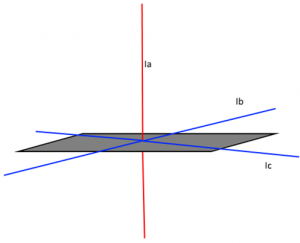# Parallel & Perpendicular Axis Theorems

## What is Parallel Axis Theorem?

Parallel axis theorem states that

The moment of inertia of a body about an axis parallel to the body passing through its center is equal to the sum of moment of inertia of body about the axis passing through the center and product of mass of the body times the square of distance between the two axes.

## Parallel Axis Theorem Formula

Parallel axis theorem statement can be expressed as follows:

 I = Ic + Mh2

Where,

• I is the moment of inertia of the body
• Ic is the moment of inertia about the center
• M is the mass of the body
• h2 is the square of distance between the two axes

## Parallel Axis Theorem Derivation

Let Ic be the moment of inertia of an axis which is passing through the center of mass (AB from the figure) and I be the moment of inertia about the axis A’B’ at a distance of h.

Consider a particle of mass m at a distance r from the center of gravity of the body.
Then,
Distance from A’B’ = r + h

I = ∑m (r + h)2

I = ∑m (r2 + h2 + 2rh)

I = ∑mr2 + ∑mh2 + ∑2rh

I = Ic + h2∑m + 2h∑mr

I = Ic + Mh2 + 0

I = Ic + Mh2

Hence, above is the formula of parallel axis theorem.

### Parallel Axis Theorem of Rod

Parallel axis theorem of rod can be determined by finding the moment of inertia of rod.
Moment of inertia of rod is given as:
I = $\frac{1}{3}$ ML2

The distance between the end of the rod and its center is given as:
h = $\frac{L}{2}$

Therefore, the parallel axis theorem of rod is:
Ic = $\frac{1}{3}$ML2 – M$\frac{L}{2}$2

Ic = $\frac{1}{3}$ML2 – $\frac{1}{4}$ML2

Ic = $\frac{1}{12}$ ML2

## Perpendicular Axis Theorem

Perpendicular axis theorem states that

For any plane body the moment of inertia about any of its axes which are perpendicular to the plane is equal to the sum of the moment of inertia about any two perpendicular axes in the plane of the body which intersect the first axis in the plane.

## Perpendicular Axis Theorem Formula

Perpendicular axis theorem is used when the body is symmetric in shape about two out of the three axes, if moment of inertia about two of the axes are known the moment of inertia about the third axis can be found using the expression:

 $I_a = I_b+I_c$Say in an engineering application we have to find the moment of inertia of a body, but the body is irregularly shaped, and the moment of in these cases we can make use of the parallel axis theorem to get the moment of inertia at any point as long as we know the center of gravity of the body, this is a very useful theorem in space physics where calculation of moment of inertia of space-crafts and satellites, making possible for us to reach the outer planets and even the deep space. The theorem of perpendicular axis helps in applications where we don’t have access to one axis of a body and it is vital for us to calculate the moment of inertia of the body in that axis.

Assignment: If the moment of inertia of a body along a perpendicular axis passing through its center of gravity is 50 kg·m2 and the mass of the body is 30 Kg. What is the moment of inertia of the same body along another axis which is 50 cm away from the current axis and parallel to it?

Solution: From parallel axis theorem,

I = $I_G~+~Mb^2$

I = 50 + ( 30 \times $0.5^2$ )

I = 57.5 kg – $m^2$